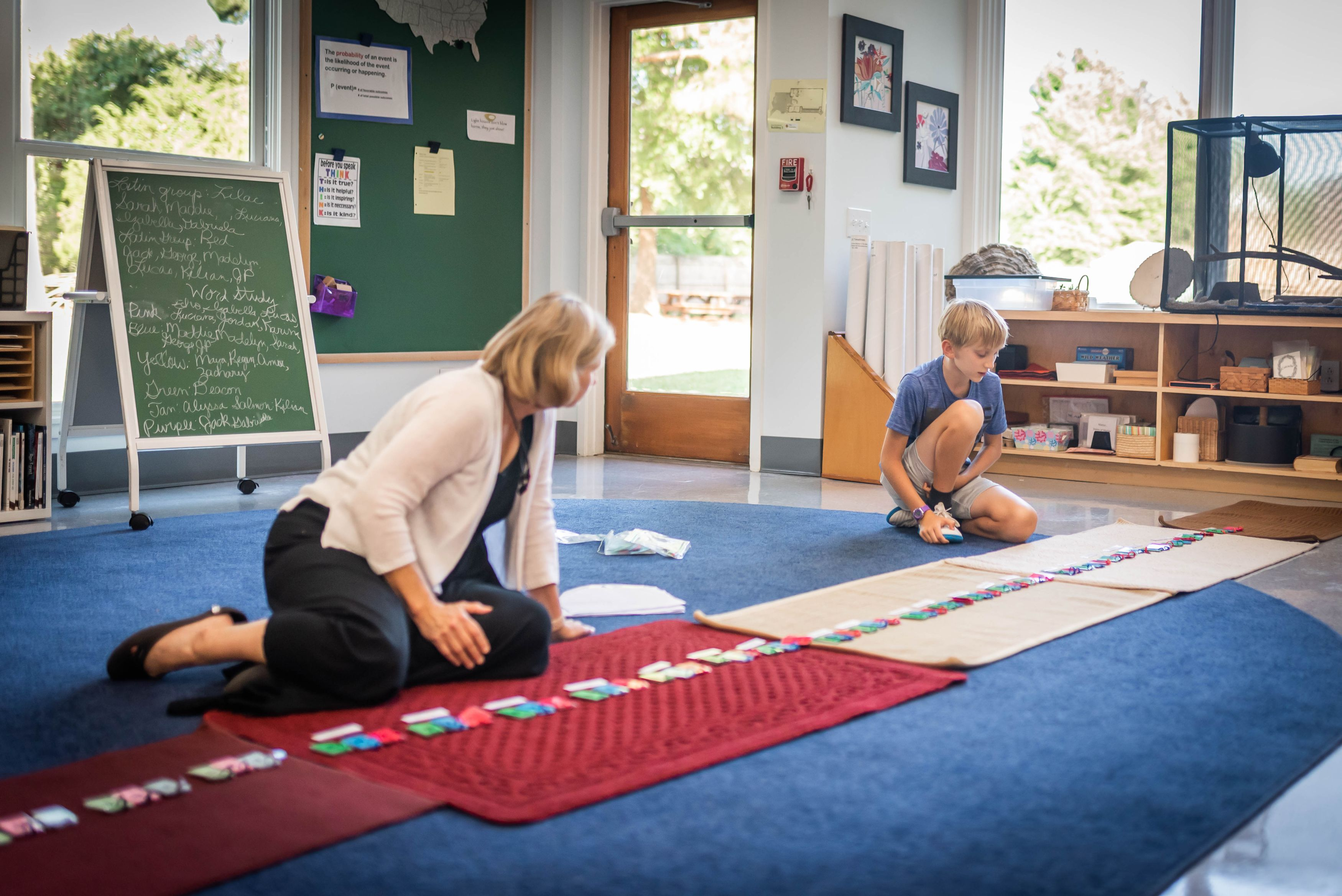Education for life

# Mathematics

Omni teaches mathematics in an authentic Montessori fashion — not as an independent and theoretical discipline, but as an aspect of culture. The Story of Numerals recounts the origins of counting, measuring, and numeric symbols. Classroom materials are three-dimensional and manipulative, helping children reveal arithmetic, geometric, and algebraic correlations - and forming meaningful images of abstract concepts. As Elementary students move out of the sensorial stage and into the development of the reasoning mind, they are no longer interested in repeating the same exercises. However, repetition is still necessary and comes through working on core skills in a variety of ways. The branches of mathematics presented in the Elementary classrooms are:

Arithmetic, starting with mathematical symbols and nomenclature, and including addition, subtraction, multiplication and division. Students gain an understanding of numeration, the decimal number system, negative numbers, fractions, and percentages.

Algebra, which is generalized arithmetic applied to unknown quantities. Students study the properties of math, non-decimal base numbers, powers of numbers, operations with exponents, square and cube roots, and powers of ten, including word problems. The Upper Elementary curriculum also includes studies with graphs, probability and statistics, algebraic expressions and equations, ratio, and rate and proportion.

Geometry, including the history of Euclid and Pythagoras. Students study nomenclature and symbols, as well lines, polygons, circles, and measurement. Guides lead the child to improve the reasoning process and understand relationships, so they can begin to calculate area, volume, and more.